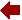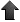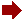HW #04
 Due: 02/13/2019
1. Obtain the first non-zero 3 terms of the Taylor series and state the convergence range for
 f(z) = z 1 − z about     z = −1.
2. Explain

 1 − 1 + 1− 1+ 1− 1+ … = 1 2
using analytic continuation.

3. Prove that
 sin(2 (x + i y)) = 2 sin (x + i y)  cos (x + i y).

File translated from TEX by TTH, version 4.03.
On 06 Feb 2019, 15:40.